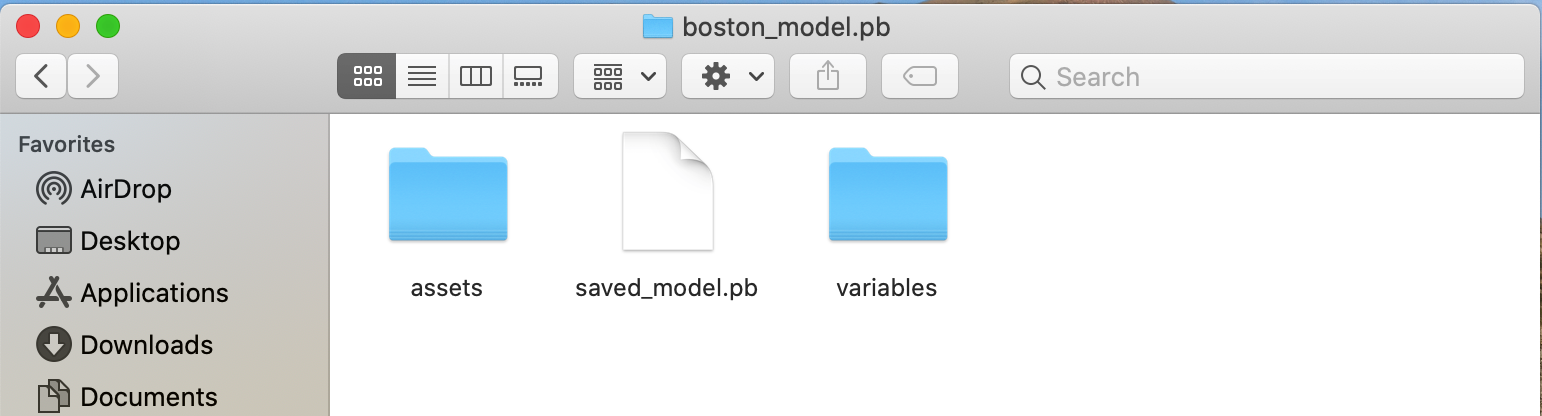2021/03/29

# 前言

Tensorflow 有三種模型儲存方式。第一種是存成 checkpoint 檔(.ckpt)，使用時機是訓練過程中欲保存目前 session 狀態。第二種是存成 pb 檔(.pb)，如果模型架構已確定或是訓練已結束，準備匯出應用時，可以直接存成 pb 檔。第三種是 Keras (目前已合併到 TF2.0) 的 `save()` 直接存成 HDF5 檔(.h5)，HDF 是設計用來儲存和組織大量資料的一組檔案格式，其內容包含了模型架構與權重。本篇文章透過波士頓房價預測資料集，訓練一個 DNN 模型並示範如何匯出與載入 `.pb``.h5` 模型檔。

## 建構模型

``````import tensorflow as tf
from tensorflow import keras
from tensorflow.keras import layers

model = keras.Sequential()
input_shape=(x_train.shape,)))
``````

``````# 編譯模型用以訓練 (設定 optimizer, loss function, metrics, 等等)

# 設定訓練參數
batch_size = 32  # 每次看 batch_size 筆的資料就更新權重
epochs = 50      # 一個 epoch 會看過一次所有的資料

# 訓練模型
model_history = model.fit(x=x_train, y=y_train,
batch_size=batch_size,
epochs=epochs,
validation_data=(x_valid, y_valid),
shuffle=True)
``````

## 儲存模型

• HDF5 檔
• pb 檔

#### HDF5 檔

``````model.save('./weights/boston_model.h5')
``````#### pb 檔

``````model.save('./checkpoints/boston_model.pb')
``````model.save 會保存網絡結構，可以直接載入並預測。 model.saveweights 只保存權重，需要重新構建網絡，才能加載權重。

## 載入模型

#### HDF5 檔

``````myModel = keras.models.load_model('./weights/boston_model.h5')
``````

#### pb 檔

``````myModel = keras.models.load_model('./checkpoints/boston_model.pb')
``````

## 故障排除

### 問題1

``````OSError: SavedModel file does not exist at: ./save/model/s/{saved_model.pbtxt|saved_model.pb}
``````
``````# 解決辦法
``````

### 問題2

``````WARNING:tensorflow:No training configuration found in save file, so the model was *not* compiled. Compile it manually.
``````

``````# 解決辦法https://www.youtube.com/channel/UCSNPCGvMYEV-yIXAVt3FA5A